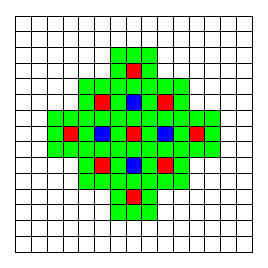### Problem Statement

We have an infinite chess board, and a new type of chess piece called a "superking". It can perform two types of moves:

• Type-1: the normal king move - i.e., a move to a neighboring square in any of the eight directions horizontal, vertical or diagonal.
• Type-2: a move two squares to the left, right, up or down.

The superking begins at some square in the infinite board and performs type1 Type-1 moves and type2 Type-2 moves. Return the number of possible squares that the superking can reach after performing these moves.

### Definition

 Class: Superking Method: squares Parameters: int, int Returns: long Method signature: long squares(int type1, int type2) (be sure your method is public)

### Constraints

-type1 and type2 will be between 0 and 100000000 (108), inclusive.

### Examples

0)

 `0` `0`
`Returns: 1`
 No moves are made, superking remains at the starting square.
1)

 `1` `0`
`Returns: 8`
 After one Type-1 move (normal king move), the superking can land in one of the 8 neighboring squares.
2)

 `0` `1`
`Returns: 4`
 After one Type-2 move, the superking lands in one of the four squares at a distance of 2 from the starting square (to the left, right, up or down).
3)

 `1` `2`
`Returns: 60`
 Let's assume that the superking starts at the red square at the center of the board shown below. After one Type-2 move, the superking can land in one of the blue squares. After the second Type-2 move, the superking can land in one of the red squares. After a final Type-1 move, the superking can land in any square neighboring a red square, i.e., one of the green squares. These are the possible final landing squares for the king after 1 Type-1 and 2 Type-2 moves, 60 in total (note that it is not important in which order we make the moves; here, we first did the two Type-2 moves and then the Type-1 move).4)

 `31` `17`
`Returns: 14713`
5)

 `24` `45`
`Returns: 35881`
6)

 `100000000` `100000000`
`Returns: 280000000400000001`
 The maximum return value.

#### Problem url:

http://www.topcoder.com/stat?c=problem_statement&pm=4836

#### Problem stats url:

http://www.topcoder.com/tc?module=ProblemDetail&rd=8096&pm=4836

gepa

#### Testers:

PabloGilberto , lbackstrom , vorthys , Olexiy

Math